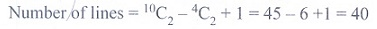# Chapter 4: Combinatorics and Mathematical Induction - Online Test

Q1. The sum of the digits at the 10th place of all numbers formed with the help of 2, 4, 5, 7 taken all at a time is
Explaination / Solution: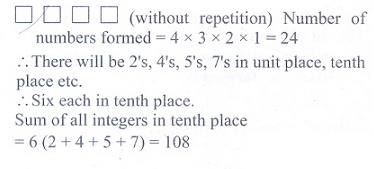Q2. In an examination there are three multiple choice questions and each question has 5 choices . Number of ways in which a student can fail to get all answer correct is
Explaination / Solution: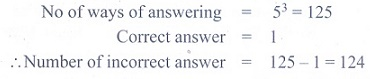Q3. The number of ways in which the following prize be given to a class of 30 boys first and second in mathematics, first and second in physics, first in chemistry and first in English is
Explaination / Solution:Q4. The number of 5 digit numbers all digits of which are odd is
Explaination / Solution: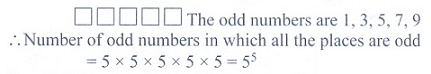Q5. In 3 fingers, the number of ways four rings can be worn is ………..ways.
Explaination / Solution:

4 rings can be worn in 3 fingers in 3ways
Q6. If (n+5)P(n+1) = ( 11(n-1) /2 )(n+3)Pn, then the value of n are
Explaination / Solution: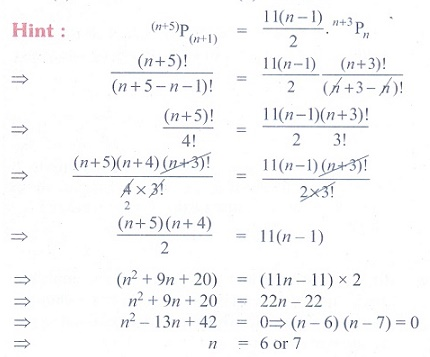Q7. The product of r consecutive positive integers is divisible by
Explaination / Solution:

Product of r consecutive positive integers is divisible by r! (theorem)
Q8. The number of five digit telephone numbers having at least one of their digits repeated is
Explaination / Solution: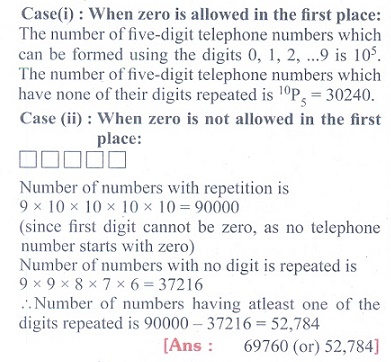Q9. If a2-aC2 = a2-a C4 then the value of ’a’ is
Explaination / Solution:Q10. There are 10 points in a plane and 4 of them are collinear. The number of straight lines joining any two points is
Explaination / Solution: